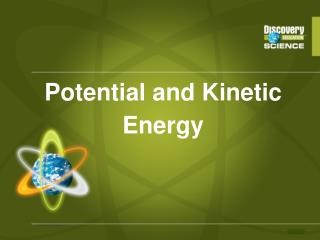DownloadDownload PresentationPotential and Kinetic Energy

# Potential and Kinetic Energy

Download Presentation## Potential and Kinetic Energy

- - - - - - - - - - - - - - - - - - - - - - - - - - - E N D - - - - - - - - - - - - - - - - - - - - - - - - - - -
##### Presentation Transcript

1. Potential and Kinetic Energy

2. Energy • Energy = the ability to do work, or move an object from one position to another • Two categories—kinetic energy and potential energy • Units for energy are Joules(J)

3. Kinetic Energy • KE = energy of an object in motion • Types of kinetic energy: • heat, • electricity, • waves (sound, EM) • the motion of objects

4. Potential Energy • PE = energy of an object due to its position, internal structure, or chemical composition • Energy that is “stored” until it can be converted into some form of kinetic energy • Types of PE include: • gravitational, • chemical, • nuclear, • elastic, • Electric

5. Equation for Potential Energy PE PE Acceleration due to gravity (9.8 m/s2 on Earth)

6. Practice Problems PE = mgh m= 5 kg g= 9.8 m/s2 h= _____ PE = ? PE = 5kg x 9.8m/s2 x 5m = 245J 5 m

7. Equation for Kinetic Energy KE KE = kinetic energy m = mass of the object v = velocity

8. Practice Problem KE = (½ )mv2 or KE = (0.5)mv2 m= 100 kg v= 10 m/s KE = ? KE= .5 x 100kg x (10m/s)2 KE= 50kg x 100m2/s2 KE= 5,000 J

9. What’s the Kinetic Energy? • What is the Kinetic Energy (in Joules) of an object with a mass of 10 kg and a velocity of 10 m/s? J

10. Different Forms of Energy • 6 different types: • Mechanical • Thermal Energy • Chemical Energy • Electrical Energy • Electromagnetic Energy • Nuclear Energy

11. Mechanical Energy • associated w/ the motion (kinetic) or position of an object (potential) • Kinetic Energy exists whenever an object which has mass is in motion with some velocity. Everything you see moving about has kinetic energy. • Potential Energy exists whenever an object which has mass has a position within a force field. The most everyday example of this is the position of objects in the earth's gravitational field. PE = Weight x Height

12. Thermal Energy • associated w/energy of the particles in an object. As thermal energy increases, the temperature of the object increases.

13. Electrical Energy • Moving electrical charges. Electricity!!

14. Electromagnetic energy • Travels in waves, associated w/ light, infrared, ultraviolet, microwaves, x-rays, etc • Longer wavelength yields low frequency & low energy • Shorter wavelength yield high frequency & high energy

15. Nuclear Energy • Associated w/ the fusion or fission of nuclear atoms. The fusion of hydrogen into helium fuels the power of the sun

16. 5.2 Energy Conversion and Conservation • Law of the Conservation of Energy - states that energy cannot be created or destroyed. It simply changes from one form into another • Most forms of energy can be converted from one type to another. • Einstein’s theory of Relativity - E = mc2 • E = the energy produced • m = the mass being converted • c = the speed of light

17. Common Misconceptions • “All energy is kinetic energy.” • Reality: There are two main classes of energy—kinetic and potential. Potential energy is the energy stored in an object.

18. Common Misconceptions • “Objects that are not moving possess no energy.” • Reality: All objects possess energy. For example, a rock lying on the ground contains a certain amount of heat energy, the kinetic energy of the molecules that make up the rock.

19. Common Misconceptions • “Gravity provides the only source of potential energy.” • Reality: There are several different forms of potential energy, including chemical, nuclear, elastic, and electric energy.

20. Common Misconceptions • “Kinetic energy only depends on speed.” • Reality: Kinetic energy also depends on an object’s mass. If two objects of different masses are traveling at the same speed, the more massive object will have more kinetic energy than the object with less mass.

21. Common Misconceptions • “Energy can be “lost” or used up.” • Reality: Energy can neither be created nor destroyed. It merely changes from one form to another. When we say that energy is “lost” during an energy transformation, what we really mean is that some of the energy is converted into heat, which is dissipated into the surroundings.